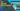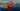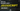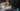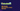# What is VLookup in Excel and How to Use it?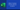Vlookup. Today we will talk about Vlookup in excel and why do we use it. Many of you who have recently started learning Microsoft Excel, are very curious about this topic.

## What is Vlookup?

Vlookup is a function in Microsoft Excel that we can use to find relevant information based on a particular field in a huge database table. This table should be organized vertically. This is why this formula is called vlookup. We also have hlookup.

Vlookup can find the data that matches Exactly as well as Approximately. It can also find the data that is partially matched.

It is like a find function that we can customise and fix it to a particular cell.

For instance, you have a large database of bank customers and you need to obtain the data of a particular account. There are numerous ways to do it but one of the ways is by applying Vlookup. Look at the screenshot below.

In the above screenshot, you can see the data of bank customers. The data has only 18 records but these records can be thousands and even millions. Imagine how difficult it would be to find out one record from 1 million records. This is when Vlookup comes in handy.

On the right-hand side of the screenshot, you can see the column names.

The idea is to just enter the account number and all the other fields Amount, Account Type, Branch, and Customer should be populated automatically by vlookup.

## VLookup Syntax

``=VLOOKUP(lookup_value,table_array,col_index_num,lookup_range)``

### Lookup_Value

You find the records based on this field. For instance bank account number.

### Table_Array

This is the data range where you look for the desired record. In the above screenshot, from Column A till Column E.

### Col_Index_Num

Column number of the desired field in table_array. For example, the column number of the amount is 2 in the above screenshot.

### Lookup_Range

This option tells the Vlookup function if you want an exact match or approximate value. We enter 0 or 1. 0 means false and 1 means true. The false means Exact match and true means Approximate match.

Now you know about the syntax and its components. It is better if you can remember the syntax. If you don’t want to remember, Microsoft Excel shows you the formula when typing.

## How to Use Vlookup?

There are two ways of implementing Vlookup.

1. Using Formula.
2. Using Wizard.

We will learn both ways in this tutorial today but let us start with the formula.

In the above screenshot, you can see the column names on the right-hand side.

## Why are We Using Vlookup?

We want to type the account number and want all the below fields self-populated just like Amount in the below screenshot. We will use the Vlookup formula in all of these cells.

## How to Write Vlookup Formula?

We will write the vlookup formula as shown underneath.

``=VLOOKUP(K1,A:E,2,0)``
1. K1 is the address of cell where Account number is saved.
2. A:E is the range of data where the vlookup will look for the desired values.
3. 2 represents the column number in A:E range.
4. 0 represents that user wants Exact Match not Approximate.

### How to Fix References in Vlookup?

#### Why do We Fix References?

Many times we write a similar formula multiple times. Let’s say three of four vlookup arguments are the same and the only difference is the column number. To save time we write the formula once and drag it.

Dragging the formula will change references. For instance, K1 becomes K2 or K3, and so on, depends how far we drag the formula. We need a way to fix the arguments so that they don’t change upon dragging.

We use the dollar sign (\$) to fix the argument. If we need to fix the K1 so we need to write it as \$K\$1. With the fixed arguments the formula will be like this.

``=VLOOKUP(\$K\$1,\$A:\$E,3,0)``

In the above formula, the first argument K1 and range A:E are fixed and will never be changed if dragged. We only need to change the column number and the formula will start showing the data from the desired column.

### Another Way to Fix the Argument

We can give names to a cell or a range of cells. Once the names are assigned, we can use the names in our formula. As you can see in the screenshot below, we have selected the cell and given a name as Account_Number. In another screenshot, we have given a name as Data_Rage to a selected range of cells.

Now we will use these names in our formula and the formula will be like this.

``=VLOOKUP(Account_Number,Data_Range,2,0)``

Now the Account_Number and Data_Range will never be changed, it does not matter how much we drag the formula.

Let us see how we will write the formula to show Amount, Account Type, Branch, and Customer.

#### Amount

``=VLOOKUP(Account_Number,Data_Range,2,0)``

#### Account Type

``=VLOOKUP(Account_Number,Data_Range,3,0)``

#### Branch

``=VLOOKUP(Account_Number,Data_Range,4,0)``

#### Customer

``=VLOOKUP(Account_Number,Data_Range,5,0)``

## Conclusion

As you can see the entire formula is the same. The only difference is the column number. This example was for the Exact match, now is the time to learn the approximate match formula.

## How to Find Approximate Value in Vlookup

Vlookup can also be used to find approximate value froom a table. Let us learn how to do it.

As we know the Vlookup function has four arguments.

1. Lookup_Value
2. Table_Array
3. Col_Index_Num
4. Lookup_Range

Lookup_Range is responsible for how the vlookup will search the data. If we do not give a Lookup_Range argument, by default it takes it as 1. 1 means approximate match. Let us see a new example to learn more.

As you can see in the example above, we have marks of the students and on the right-hand side, we have a grades table. We need to match the marks against the grade table and find the appropriate grade. Let us do it.

``=VLOOKUP(C2,\$F\$1:\$G\$6,2,1)``
1. C2 is the address of marks. As you can see in the formula, C2 is not fixed because when we will drag the formula it will change itself.
2. \$F\$1:\$G\$6 is the grade table range. We have fixed the grade table range by dollar signs.
3. 2 is the second column in the table. We have selected the second column from the grade table.
4. 1 is the sign of approximate match in this formula.

## Conclusion

As you can see, the formula is comparing the marks of the students against the grade table. The formula is not looking for an exact match but Whatever the nearest record to the student marks, that grade gets populated because we have mentioned in the formula that we want the second column from the grade table.

## How to Configure Vlookup for Wildcard Match (Partial Match)

In this example, we want to type the name in the find cell and want the matching fields self populated. Let us write the formula for Wildcard Matching.

``=VLOOKUP(\$I\$1 &  "*",\$A:\$E,1,0)``

Pay attention to the vlookup formula. We have divided the first argument into two parts. First, we used & sign to include the second part of the argument. “*” is the second part. The formula is written for an exact match with wildcard functionality.

With the first argument \$I\$1 & “*” the \$I\$1 acts when something matches. The “*” acts to include anything. Together with \$I\$1 & “*” means if a few characters of the words match and a few don’t match, the formula will ignore what is not matching and show the results for what is matching.

For example, if we just type the letter P in the find box, the formula takes Paul Jones into account because that is the first result that comes with P.

If we type Ph then the formula will take Phillip Morgan into account.

## The Trick to Make Vlookup Taking Multiple Criteria

Be informed that vlookup takes only one criteria natively this is why we need to use a trick. Using this trick we can make vlookup taking as many criteras as possible. So let us begin.

As we know vlookup takes the criteria from only one cell and based on that criterion it searches the data in the data range.

We will first go ahead and concatenate multiple criteria in a single cell. After that, we will use this single cell to identify the data. We can hide this concatenated column so that it is not shown because with 3 values inside that cell looks weird.

``=B2&" "&C2&" "&D2``

As you can see we have column A in the sheet with a formula that concatenates 3 cells B C and D. This is needed because we want a cell that has the combination of the first name, last name, and department.

We can see in this example we want to take J1, J2, and J3 as the first argument. We will do exactly that and concatenate these 3 cells with & sign. Let us do it.

``=VLOOKUP(\$J\$1&" "&\$J\$2&" "&\$J\$3,\$A\$1:\$F\$12,5,0)``

As we can see in the formula, we have taken J1, J2, and J3 as the first argument and then fixed them with the \$ sign so that they dont change.

When we selected the Table_Array, we included column A in the range. We included it because J1, J2, and J3 make a combination and that combination is in column A. When vlookup will search the combination in the range, it will find it in the first column A and it will select that record.

## How to Hide a Column

Just like the column in the example above, multiple criteria example, some columns we create in the sheet for the calculation purposes or for tricks. We can hide these columns as well by right-clicking on the column and use the option hide cell. Look at the screenshot below.

## How to Unhide a Column

To unhide a column we need to follow the steps below.

Home Tab > Format > Hide & Unhide > Unhide Column

## Two Way Vlookup

Before we start talking about Two Way Vlookup, we much know about Match Function in Excel.

### Match Function

The Match function takes 3 arguments. Lookup value, Lookup range, and Match type (Exact Match, or Approximate Match) then returns the position of that lookup value.

``MATCH(lookup_value, lookup_array, [match_type])``

We can use the Match function in vlookup where vlookup asks for col_index_num. We will provide the Match function the address of a lookup value just like we do with vlookup. This way we can change the column inside the formula without changing the formula.

Now that we know about Match Function, let’s start Two Way Vlookup. So far we have learned, the vlookup takes 4 arguments. The third argument Col_Index_Num is always hard-coded.

What if we make Col_Index_Num dynamic? we will have a dynamic two-way vlookup. Where we can change both column and row in the same formula. Let us see an example.

### Example-

Suppose we have a list of medicines with how much each medicine sold in each month. The data will look like this.

Now lets see how can we write the formula for two way vlookup.

``=VLOOKUP(P2,A1:M11,MATCH(P1,A1:M1,0),0)``

As you can see we have used a Match function of Microsoft Excel in vlookup. The vlookup is providing the col_index_num to vlookup. Let us look at the outcome in the below screenshot.

In the sheet, you can see we have two arguments in the P2 and P2. P1 is for Vlookup and P2 is for Match formula inside vlookup. In the third cell, the vlookup is giving us desired value according to P1 and P2. Because vlookup is taking row as vlookup value and column are taking from Match lookup value. This is why it’s called Two Way Vlookup.

## How to Deal with #N/A Errors

The error #N/A simply means Not Applicable. We get this error when the formula does not get the matching data. We have ways to change or hide this error message. The best way is to use the IFNA formula.

### Using IFNA Formula

#### To Hide the #N/A Errors

``=IFNA(VLOOKUP(\$I\$1 &  "*",\$A:\$E,1,0),"")``

#### To Change the #N/A Errors

``=IFNA(VLOOKUP(\$I\$1 &  "*",\$A:\$E,1,0),"Data not found")``

As you can see in the screenshots above, we can use the IFNA function and edit and hide the #N/A Error. You can make it whatever you want.

## Using IFERROR Formula

The IFNA was the first way to deal with #N/A error because that formula is specifically designed to deal with #N/A. We can also use IFERROR formula. This handles any type of error.

``=IFERROR(VLOOKUP(\$I\$1 &  "*",\$A:\$E,1,0),"Data not found")``

The syntax of the IFERROR is same as IFNA. We can make use of either of them.

I hope the article was helpful and you have understood how to use vlookup. Please comment for any feedback or confusion. Here is a file that you can download to practice vlookup.

Article Suggestion – How to Remove Duplicates from Microsoft Excel Sheet.

Tags

Search by